Started ‎09-02-2022 by
Modified ‎09-02-2022 by
Views 565

Beginning in Stable release 2022.1.1 (May 2022), you can download models from the Interactive Modeling Node in Visual Forecasting and then test the downloaded code in SAS Studio. The documentation illustrates this using KUBECTL commands via a Linux shell. Because many Visual Forecasting users are more comfortable staying in the SAS tools rather than jumping to a Linux shell, I demonstrate in this blog how to accomplish the same thing using the power of SAS Visual Analytics, SAS Model Studio, and SAS Studio.

For this example, I am using Stable release 2022.1.2.  I'll use electricity generation data from the US Energy Information Administration with roles assigned as follows.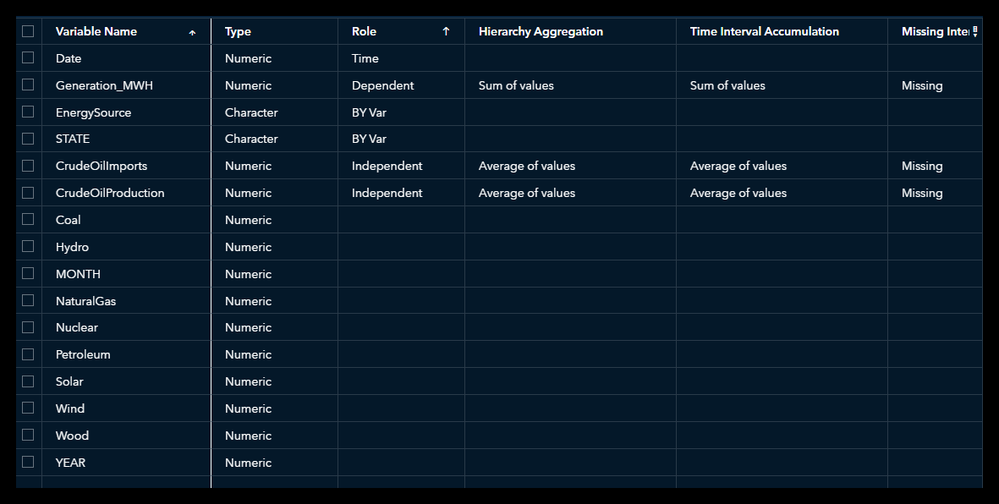Select any image to see a larger version.
Mobile users: To view the images, select the "Full" version at the bottom of the page.

Under nodes, notice that the miscellaneous category and Save Data are new in Stable 2022.1.2.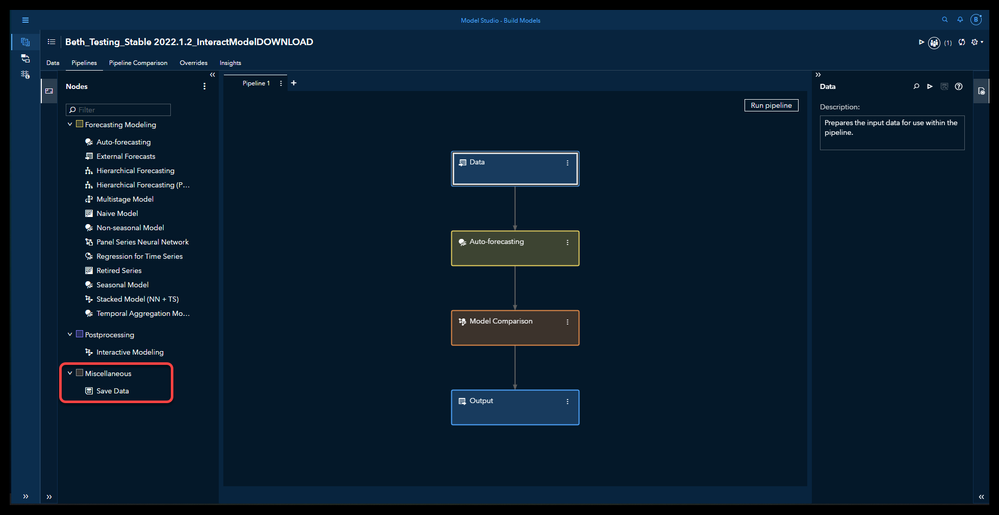Add the interactive node after the Auto-forecasting node and run the pipeline.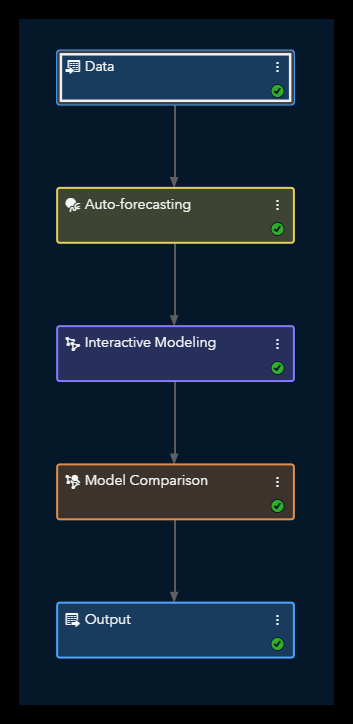Right click the Interactive Modeling node and select Open.It should open on the Modeling tab. If not, go to the Modeling tab.Select a single time series. For this example, I’ve selected Wind:CA.  Select the model you want to download (here DIAG1_ARIMAX1), right click, download.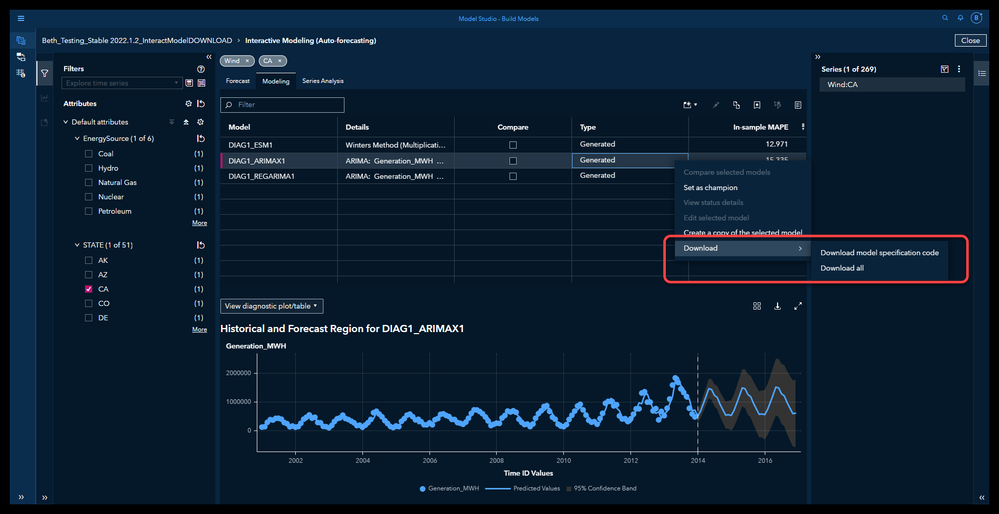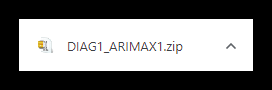Extract these files to a location available to you from your local client. For simplicity’s sake we are using a model without events, so there are only 2 .csv files downloaded:

• seriesData.csv
• seriesColumnInfo.csv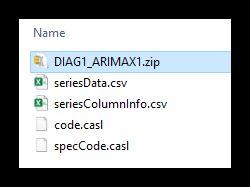Open Visual Analytics (Explore and Visualize).  Select Start with Data, Import.  Navigate to your csv files seriesColumnInfo.csv and seriesData.csv and import each of them. By default they will import into cas-shared-default/Public and sashdat files.Use the hamburger icon at the top left to open the applications menu and select Develop SAS Code to open SAS Studio.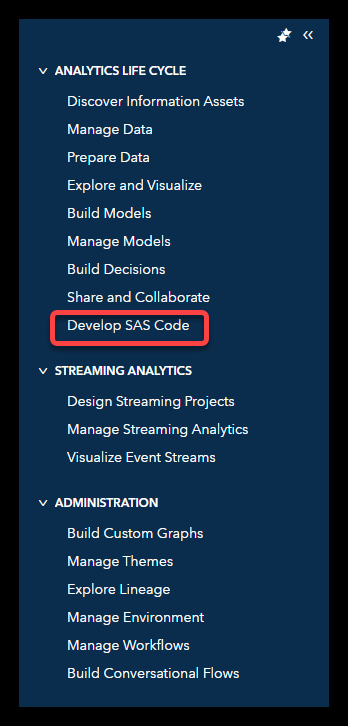Copy the contents of code.casl into SAS Studio. Replace the beginning of the code with:

``````/* Create a CAS session and a CAS library */
cas mycas sessopts=(caslib=”public” timeout=1800 locale=”en_US”);
libname mylib cas;

/* Start CAS Procedure */
proc cas;
seriesTableCaslib = “PUBLIC”; /* CASLIB where input tables have been loaded. */
seriesTableName = “seriesData”; /* Name of the loaded CAS table for the time series data. */
seriesTableMap = “seriesColumnInfo”; /* Name of the loaded CAS table for the time series table’s column information. */
outTablePrefix = “DIAG1_ARIMAX1.”; /* Prefix for the generated output tables. */
outTableCaslib = “PUBLIC”; /* CASLIB where output tables should be generated. */
existCondition = true;``````

Delete all the code having to do with loading the .csv files as shown in strikeout below.  You don't need this code because you have already loaded the files using the SAS Visual Analytics visual interface . Do not delete the timeData.runTimeCode statement or any statements beyond that.

ccc

/* Do not modify anything below this line without fully understanding the code. */

function getAttributes(tableColumnInfo, caslib);

/* Load the series columnInformation. */

casOut = {name=tableColumnInfo, caslib=caslib, replace=true}

path = csvPath || tableColumnInfo || “.csv”

importOptions = {fileType=”csv”, varChars=false};

table.fetch result = r / table={name=tableColumnInfo, caslib=caslib}

index = false

sortBy = “id”

to = 2147483647

maxRows = 2147483647;

varsAttributes[dim(r.fetch)] = {}; i = 1;

do row over r.fetch;

thisVar = {name=trim(row.column), type=trim(row.type), formattedLength=row.formattedLength,

nfl=row.nfl, nfd=row.nfd};

if upcase(trim(row.type)) eq “CHAR” then thisVar = thisVar + {length=row.rawLength};

if trim(row.format) ne “” then thisVar = thisVar + {format=row.format};

if trim(row.label) ne “” then thisVar = thisVar + {label=trim(row.label)}; varsAttributes[i] = thisVar; i = i + 1;

end;

return varsAttributes;

end func;

/* List all files in the caslib’s data source. */

table.fileInfo result=r /

caslib = seriesTableCaslib

path = csvPath;

fileExists = {}; do file over {seriesTableName, seriesTableMap}; fileExists[file] = ((file || “.csv”) in r.FileInfo[, “name”]);

end;

if fileExists[seriesTableName] then do;

if fileExists[seriesTableMap] then varsAttributes = getAttributes(seriesTableMap, seriesTableCaslib);

else do;

fileName = seriesTableMap;

existCondition = false;

goto doneViewCode;

end;

/* Load the series data. */

casOut = {name=seriesTableName, caslib=seriesTableCaslib, replace=true}

path = csvPath || seriesTableName || “.csv”

importOptions = {fileType=”csv”, varchars=false, vars=varsAttributes};

end;

else do;

fileName = seriesTableName;

existCondition = false;

goto doneViewCode;

end;

Leave all the code beginning with timeData.runTimeCode as shown in the example code below.

``````timeData.runTimeCode /
table = {name=seriesTableName, caslib=seriesTableCaslib},
timeId = {name=”Date”},
interval = “month”,
trimId = “left”,
require = {{pkg=”atsm”}, {pkg=”tsm”}},
seasonality = 12,
objOut = {
{table = {name=outTablePrefix || “OUTFOR”, caslib=outTableCaslib, replace=true}, objRef=”outFor”},
{table = {name=outTablePrefix || “OUTSTAT”, caslib=outTableCaslib, replace=true}, objRef=”outStat”},
{table = {name=outTablePrefix || “OUTSELECT”, caslib=outTableCaslib, replace=true}, objRef=”outSelect”},
{table = {name=outTablePrefix || “OUTMODELINFO”, caslib=outTableCaslib, replace=true}, objRef=”outModelInfo”},
{table = {name=outTablePrefix || “OUTFMSG”, caslib=outTableCaslib, replace=true}, objRef=”outFmsg”},
{table = {name=outTablePrefix || “OUTEST”, caslib=outTableCaslib, replace=true}, objRef=”outEst”}
},
logControl = {{sev=”error”, keep=true}, {sev=”warning”, keep=true}, {sev=”note”, keep=true}},
errorStop = true,
logOut = {name=outTablePrefix || “OUTLOG”, caslib=outTableCaslib, replace=true},
series = {{name=”Generation_MWH”, accumulate=”sum”, setMiss=”missing”},
{name=”CrudeOilProduction”, accumulate=”avg”, setMiss=”missing”},
{name=”CrudeOilImports”, accumulate=”avg”, setMiss=”missing”}},
code = ‘
declare object dataFrame(tsdf);
rc = dataFrame.Initialize();
rc = dataFrame.AddX(“CrudeOilProduction”n, “required”, “yes”, “extend”, “stochastic”);
rc = dataFrame.AddX(“CrudeOilImports”n, “required”, “yes”, “extend”, “stochastic”);

/* Generate the ARIMA specifications for the model DIAG1_ARIMAX1. */
declare object DIAG1_ARIMAX1(arimaspec);
rc = DIAG1_ARIMAX1.Open();
/* Add the moving average (MA) polynomial factor 1. */
array ma_1_1/nosymbols;
ma_1_1 = 1;
/* Add the seasonal moving average (MA) polynomial factor 1. */
array mas_1_1/nosymbols;
mas_1_1 = 1;
array diff_1/nosymbols;
diff_1 = 1;
diff_1 = 12;
rc = DIAG1_ARIMAX1.SetDiff(diff_1);
rc = DIAG1_ARIMAX1.SetOption(“NOINT”,1);
/* Add the functional transform none with inverse transform none. */
rc = DIAG1_ARIMAX1.SetTransform(“none”, “none”);
/* Add the input in the form of a transfer function. */
array inputDiffArray_1_1/nosymbols;
inputDiffArray_1_1 = 1;
inputDiffArray_1_1 = 12;
rc = DIAG1_ARIMAX1.SetTFTransform(“CrudeOilProduction”, “none”);
/* Add the input in the form of a transfer function. */
array inputDiffArray_1_2/nosymbols;
inputDiffArray_1_2 = 1;
inputDiffArray_1_2 = 12;
rc = DIAG1_ARIMAX1.SetTFTransform(“CrudeOilImports”, “none”);
/* Add the estimation settings, if there are any. */
rc = DIAG1_ARIMAX1.SetOption(“method”, “cls”);
rc = DIAG1_ARIMAX1.SetOption(“converge”, 0.001);
rc = DIAG1_ARIMAX1.SetOption(“maxiter”, 50);
rc = DIAG1_ARIMAX1.SetOption(“delta”, 0.001);
rc = DIAG1_ARIMAX1.SetOption(“singular”, 1E-7);
rc = DIAG1_ARIMAX1.SetOption(“nostable”, 0);
rc = DIAG1_ARIMAX1.Close();

declare object forecast(foreng);
rc = forecast.Initialize(dataFrame);
rc = forecast.SetOption(“minobs.trend”, 2);
rc = forecast.SetOption(“minobs.season”, 2);
rc = forecast.SetOption(“holdout”, 0);
rc = forecast.SetOption(“holdoutPct”, .);
rc = forecast.SetOption(“minobs.mean”, 2);
rc = forecast.SetOption(“alpha”, 0.05);
rc = forecast.SetOption(“horizon”, 19724);
rc = forecast.SetOption(“back”, 0);
rc = forecast.SetOption(“fcst.bd.lower”, 0);
rc = forecast.SetOption(“noaltlist”, 1);
rc = forecast.Run();

declare object outFor(outFor);
declare object outStat(outStat);
declare object outSelect(outSelect);
declare object outModelInfo(outModelInfo);
declare object outFmsg(outFmsg);
declare object outEst(outEst);
rc = outFor.Collect(forecast);
rc = outStat.Collect(forecast);
rc = outSelect.Collect(forecast);
rc = outModelInfo.Collect(forecast);
rc = outFmsg.Collect(forecast);
rc = outEst.Collect(forecast);
‘;

doneViewCode:
if existCondition eq false then do;
print (error) fileName || “.csv is not accessible at the specificed path.”;
end;``````

Run the code. Ta dah! Your results will look something like this.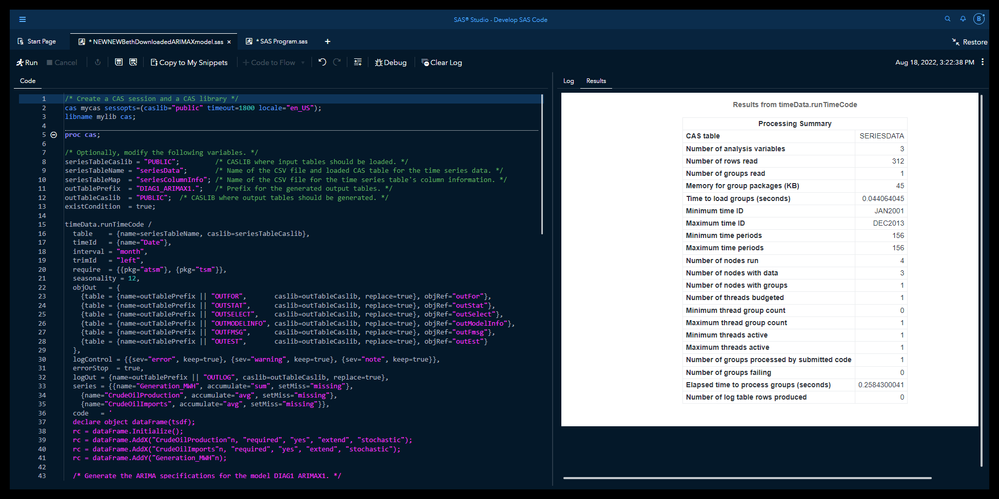You might have many reasons for downloading your models and data.  Now you see how easy it is to download models and data as .csv files from SAS Visual Forecasting using SAS Model Studio, loading any needed data into memory using SAS Visual Analytics, and running or testing the code in SAS Studio.

Find more articles from SAS Global Enablement and Learning here.

Version history
Last update:
‎09-02-2022 01:10 PM
Updated by:Contributors
Article Labels
Article Tags